# Metrics#

All high-level functions like HindcastEnsemble.verify(), HindcastEnsemble.bootstrap(), PerfectModelEnsemble.verify() and PerfectModelEnsemble.bootstrap() have a metric argument that has to be called to determine which metric is used in computing predictability.

Note

We use the term ‘observations’ o here to refer to the ‘truth’ data to which we compare the forecast f. These metrics can also be applied relative to a control simulation, reconstruction, observations, etc. This would just change the resulting score from quantifying skill to quantifying potential predictability.

Internally, all metric functions require forecast and observations as inputs. The dimension dim has to be set to specify over which dimensions the metric is applied and are hence reduced. See Comparisons for more on the dim argument.

## Deterministic#

Deterministic metrics assess the forecast as a definite prediction of the future, rather than in terms of probabilities. Another way to look at deterministic metrics is that they are a special case of probabilistic metrics where a value of one is assigned to one category and zero to all others .

### Correlation Metrics#

The below metrics rely fundamentally on correlations in their computation. In the literature, correlation metrics are typically referred to as the Anomaly Correlation Coefficient (ACC). This implies that anomalies in the forecast and observations are being correlated. Typically, this is computed using the linear Pearson Product-Moment Correlation. However, climpred also offers the Spearman’s Rank Correlation.

Note that the p value associated with these correlations is computed via a separate metric. Use _pearson_r_p_value() or _spearman_r_p_value() to compute p values assuming that all samples in the correlated time series are independent. Use _pearson_r_eff_p_value() or _spearman_r_eff_p_value() to account for autocorrelation in the time series by calculating the _effective_sample_size().

#### Pearson Product-Moment Correlation Coefficient#

# Enter any of the below keywords in metric=... for the compute functions.
In : print(f"Keywords: {metric_aliases['pearson_r']}")
Keywords: ['pearson_r', 'pr', 'acc', 'pacc']

climpred.metrics._pearson_r(forecast: xarray.Dataset, verif: xarray.Dataset, dim: Optional[Union[str, List[str]]] = None, **metric_kwargs: Any) [source]#

Pearson product-moment correlation coefficient.

A measure of the linear association between the forecast and verification data that is independent of the mean and variance of the individual distributions. This is also known as the Anomaly Correlation Coefficient (ACC) when correlating anomalies.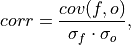where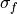and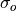represent the standard deviation of the forecast and verification data over the experimental period, respectively.

Note

Use metric _pearson_r_p_value() or _pearson_r_eff_p_value() to get the corresponding p value.

Parameters

Notes

 minimum -1.0 maximum 1.0 perfect 1.0 orientation positive

Example

>>> HindcastEnsemble.verify(
...     metric="pearson_r",
...     comparison="e2o",
...     alignment="same_verifs",
...     dim=["init"],
... )
<xarray.Dataset>
Coordinates:
* lead     (lead) int32 1 2 3 4 5 6 7 8 9 10
skill    <U11 'initialized'
Data variables:
SST      (lead) float64 0.9272 0.9145 0.9127 0.9319 ... 0.9315 0.9185 0.9112
Attributes:
skill_calculated_by_function:  HindcastEnsemble.verify()
number_of_initializations:     64
number_of_members:             10
alignment:                     same_verifs
metric:                        pearson_r
comparison:                    e2o
dim:                           init
reference:                     []


#### Pearson Correlation p value#

# Enter any of the below keywords in metric=... for the compute functions.
In : print(f"Keywords: {metric_aliases['pearson_r_p_value']}")
Keywords: ['pearson_r_p_value', 'p_pval', 'pvalue', 'pval']

climpred.metrics._pearson_r_p_value(forecast: xarray.Dataset, verif: xarray.Dataset, dim: Optional[Union[str, List[str]]] = None, **metric_kwargs: Any) [source]#

Probability that forecast and verification data are linearly uncorrelated.

Two-tailed p value associated with the Pearson product-moment correlation coefficient _pearson_r(), assuming that all samples are independent. Use _pearson_r_eff_p_value() to account for autocorrelation in the forecast and verification data.

Parameters

Notes

 minimum 0.0 maximum 1.0 perfect 1.0 orientation negative

Example

>>> HindcastEnsemble.verify(
...     metric="pearson_r_p_value",
...     comparison="e2o",
...     alignment="same_verifs",
...     dim="init",
... )
<xarray.Dataset>
Coordinates:
* lead     (lead) int32 1 2 3 4 5 6 7 8 9 10
skill    <U11 'initialized'
Data variables:
SST      (lead) float64 5.779e-23 2.753e-21 4.477e-21 ... 8.7e-22 6.781e-21
Attributes:
skill_calculated_by_function:  HindcastEnsemble.verify()
number_of_initializations:     64
number_of_members:             10
alignment:                     same_verifs
metric:                        pearson_r_p_value
comparison:                    e2o
dim:                           init
reference:                     []


#### Effective Sample Size#

# Enter any of the below keywords in metric=... for the compute functions.
In : print(f"Keywords: {metric_aliases['effective_sample_size']}")
Keywords: ['effective_sample_size', 'n_eff', 'eff_n']

climpred.metrics._effective_sample_size(forecast: xarray.Dataset, verif: xarray.Dataset, dim: Optional[Union[str, List[str]]] = None, **metric_kwargs: Any) [source]#

Effective sample size for temporally correlated data.

Note

Weights are not included here due to the dependence on temporal autocorrelation.

Note

This metric can only be used for hindcast-type simulations.

The effective sample size extracts the number of independent samples between two time series being correlated. This is derived by assessing the magnitude of the lag-1 autocorrelation coefficient in each of the time series being correlated. A higher autocorrelation induces a lower effective sample size which raises the correlation coefficient for a given p value.

The effective sample size is used in computing the effective p value. See _pearson_r_eff_p_value() and _spearman_r_eff_p_value().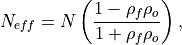where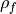and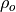are the lag-1 autocorrelation coefficients for the forecast and verification data.

Parameters

Notes

 minimum 0.0 maximum ∞ perfect N/A orientation positive

References

Bretherton et al. 

Example

>>> HindcastEnsemble.verify(
...     metric="effective_sample_size",
...     comparison="e2o",
...     alignment="same_verifs",
...     dim="init",
... )
<xarray.Dataset>
Coordinates:
* lead     (lead) int32 1 2 3 4 5 6 7 8 9 10
skill    <U11 'initialized'
Data variables:
SST      (lead) float64 5.0 4.0 3.0 3.0 3.0 3.0 3.0 3.0 3.0 3.0
Attributes:
skill_calculated_by_function:  HindcastEnsemble.verify()
number_of_initializations:     64
number_of_members:             10
alignment:                     same_verifs
metric:                        effective_sample_size
comparison:                    e2o
dim:                           init
reference:                     []


#### Pearson Correlation Effective p value#

# Enter any of the below keywords in metric=... for the compute functions.
In : print(f"Keywords: {metric_aliases['pearson_r_eff_p_value']}")
Keywords: ['pearson_r_eff_p_value', 'p_pval_eff', 'pvalue_eff', 'pval_eff']

climpred.metrics._pearson_r_eff_p_value(forecast: xarray.Dataset, verif: xarray.Dataset, dim: Optional[Union[str, List[str]]] = None, **metric_kwargs: Any) [source]#

pearson_r_p_value accounting for autocorrelation.

Note

Weights are not included here due to the dependence on temporal autocorrelation.

Note

This metric can only be used for hindcast-type simulations.

The effective p value is computed by replacing the sample size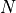in the t-statistic with the effective sample size,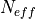. The same Pearson product-moment correlation coefficient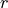is used as when computing the standard p value.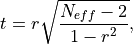whereis computed via the autocorrelation in the forecast and verification data.whereandare the lag-1 autocorrelation coefficients for the forecast and verification data.

Parameters

Notes

 minimum 0.0 maximum 1.0 perfect 1.0 orientation negative

Example

>>> HindcastEnsemble.verify(
...     metric="pearson_r_eff_p_value",
...     comparison="e2o",
...     alignment="same_verifs",
...     dim="init",
... )
<xarray.Dataset>
Coordinates:
* lead     (lead) int32 1 2 3 4 5 6 7 8 9 10
skill    <U11 'initialized'
Data variables:
SST      (lead) float64 0.02333 0.08552 0.2679 ... 0.2369 0.2588 0.2703
Attributes:
skill_calculated_by_function:  HindcastEnsemble.verify()
number_of_initializations:     64
number_of_members:             10
alignment:                     same_verifs
metric:                        pearson_r_eff_p_value
comparison:                    e2o
dim:                           init
reference:                     []


References

Bretherton et al. 

#### Spearman’s Rank Correlation Coefficient#

# Enter any of the below keywords in metric=... for the compute functions.
In : print(f"Keywords: {metric_aliases['spearman_r']}")
Keywords: ['spearman_r', 'sacc', 'sr']

climpred.metrics._spearman_r(forecast: xarray.Dataset, verif: xarray.Dataset, dim: Optional[Union[str, List[str]]] = None, **metric_kwargs: Any) [source]#

Spearman’s rank correlation coefficient.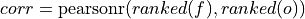This correlation coefficient is nonparametric and assesses how well the relationship between the forecast and verification data can be described using a monotonic function. It is computed by first ranking the forecasts and verification data, and then correlating those ranks using the _pearson_r() correlation.

This is also known as the anomaly correlation coefficient (ACC) when comparing anomalies, although the Pearson product-moment correlation coefficient _pearson_r() is typically used when computing the ACC.

Note

Use metric _spearman_r_p_value() or _spearman_r_eff_p_value() to get the corresponding p value.

Parameters

Notes

 minimum -1.0 maximum 1.0 perfect 1.0 orientation positive

Example

>>> HindcastEnsemble.verify(
...     metric="spearman_r",
...     comparison="e2o",
...     alignment="same_verifs",
...     dim="init",
... )
<xarray.Dataset>
Coordinates:
* lead     (lead) int32 1 2 3 4 5 6 7 8 9 10
skill    <U11 'initialized'
Data variables:
SST      (lead) float64 0.9336 0.9311 0.9293 0.9474 ... 0.9465 0.9346 0.9328
Attributes:
skill_calculated_by_function:  HindcastEnsemble.verify()
number_of_initializations:     64
number_of_members:             10
alignment:                     same_verifs
metric:                        spearman_r
comparison:                    e2o
dim:                           init
reference:                     []


#### Spearman’s Rank Correlation Coefficient p value#

# Enter any of the below keywords in metric=... for the compute functions.
In : print(f"Keywords: {metric_aliases['spearman_r_p_value']}")
Keywords: ['spearman_r_p_value', 's_pval', 'spvalue', 'spval']

climpred.metrics._spearman_r_p_value(forecast: xarray.Dataset, verif: xarray.Dataset, dim: Optional[Union[str, List[str]]] = None, **metric_kwargs: Any) [source]#

Probability that forecast and verification data are monotonically uncorrelated.

Two-tailed p value associated with the Spearman’s rank correlation coefficient _spearman_r(), assuming that all samples are independent. Use _spearman_r_eff_p_value() to account for autocorrelation in the forecast and verification data.

Parameters

Notes

 minimum 0.0 maximum 1.0 perfect 1.0 orientation negative

Example

>>> HindcastEnsemble.verify(
...     metric="spearman_r_p_value",
...     comparison="e2o",
...     alignment="same_verifs",
...     dim="init",
... )
<xarray.Dataset>
Coordinates:
* lead     (lead) int32 1 2 3 4 5 6 7 8 9 10
skill    <U11 'initialized'
Data variables:
SST      (lead) float64 6.248e-24 1.515e-23 ... 4.288e-24 8.254e-24
Attributes:
skill_calculated_by_function:  HindcastEnsemble.verify()
number_of_initializations:     64
number_of_members:             10
alignment:                     same_verifs
metric:                        spearman_r_p_value
comparison:                    e2o
dim:                           init
reference:                     []


#### Spearman’s Rank Correlation Effective p value#

# Enter any of the below keywords in metric=... for the compute functions.
In : print(f"Keywords: {metric_aliases['spearman_r_eff_p_value']}")
Keywords: ['spearman_r_eff_p_value', 's_pval_eff', 'spvalue_eff', 'spval_eff']

climpred.metrics._spearman_r_eff_p_value(forecast: xarray.Dataset, verif: xarray.Dataset, dim: Optional[Union[str, List[str]]] = None, **metric_kwargs: Any) [source]#

_spearman_r_p_value accounting for autocorrelation.

Note

Weights are not included here due to the dependence on temporal autocorrelation.

Note

This metric can only be used for hindcast-type simulations.

The effective p value is computed by replacing the sample sizein the t-statistic with the effective sample size,. The same Spearman’s rank correlation coefficientis used as when computing the standard p value.whereis computed via the autocorrelation in the forecast and verification data.whereandare the lag-1 autocorrelation coefficients for the forecast and verification data.

Parameters

Notes

 minimum 0.0 maximum 1.0 perfect 1.0 orientation negative

References

Bretherton et al. 

Example

>>> HindcastEnsemble.verify(
...     metric="spearman_r_eff_p_value",
...     comparison="e2o",
...     alignment="same_verifs",
...     dim="init",
... )
<xarray.Dataset>
Coordinates:
* lead     (lead) int32 1 2 3 4 5 6 7 8 9 10
skill    <U11 'initialized'
Data variables:
SST      (lead) float64 0.02034 0.0689 0.2408 ... 0.2092 0.2315 0.2347
Attributes:
skill_calculated_by_function:  HindcastEnsemble.verify()
number_of_initializations:     64
number_of_members:             10
alignment:                     same_verifs
metric:                        spearman_r_eff_p_value
comparison:                    e2o
dim:                           init
reference:                     []


### Distance Metrics#

This class of metrics simply measures the distance (or difference) between forecasted values and observed values.

#### Mean Squared Error (MSE)#

# Enter any of the below keywords in metric=... for the compute functions.
In : print(f"Keywords: {metric_aliases['mse']}")
Keywords: ['mse']

climpred.metrics._mse(forecast: xarray.Dataset, verif: xarray.Dataset, dim: Optional[Union[str, List[str]]] = None, **metric_kwargs: Any) [source]#

Mean Sqaure Error (MSE).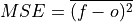The average of the squared difference between forecasts and verification data. This incorporates both the variance and bias of the estimator. Because the error is squared, it is more sensitive to large forecast errors than mae, and thus a more conservative metric. For example, a single error of 2°C counts the same as two 1°C errors when using mae. On the other hand, the 2°C error counts double for mse. See Jolliffe and Stephenson, 2011.

Parameters

Notes

 minimum 0.0 maximum ∞ perfect 0.0 orientation negative

References

Jolliffe and Stephenson 

Example

>>> HindcastEnsemble.verify(
...     metric="mse", comparison="e2o", alignment="same_verifs", dim="init"
... )
<xarray.Dataset>
Coordinates:
* lead     (lead) int32 1 2 3 4 5 6 7 8 9 10
skill    <U11 'initialized'
Data variables:
SST      (lead) float64 0.006202 0.006536 0.007771 ... 0.02417 0.02769
Attributes:
skill_calculated_by_function:  HindcastEnsemble.verify()
number_of_initializations:     64
number_of_members:             10
alignment:                     same_verifs
metric:                        mse
comparison:                    e2o
dim:                           init
reference:                     []


#### Root Mean Square Error (RMSE)#

# Enter any of the below keywords in metric=... for the compute functions.
In : print(f"Keywords: {metric_aliases['rmse']}")
Keywords: ['rmse']

climpred.metrics._rmse(forecast: xarray.Dataset, verif: xarray.Dataset, dim: Optional[Union[str, List[str]]] = None, **metric_kwargs: Any) [source]#

Root Mean Sqaure Error (RMSE).The square root of the average of the squared differences between forecasts and verification data.

Parameters

Notes

 minimum 0.0 maximum ∞ perfect 0.0 orientation negative

Example

>>> HindcastEnsemble.verify(
...     metric="rmse", comparison="e2o", alignment="same_verifs", dim="init"
... )
<xarray.Dataset>
Coordinates:
* lead     (lead) int32 1 2 3 4 5 6 7 8 9 10
skill    <U11 'initialized'
Data variables:
SST      (lead) float64 0.07875 0.08085 0.08815 ... 0.1371 0.1555 0.1664
Attributes:
skill_calculated_by_function:  HindcastEnsemble.verify()
number_of_initializations:     64
number_of_members:             10
alignment:                     same_verifs
metric:                        rmse
comparison:                    e2o
dim:                           init
reference:                     []


#### Mean Absolute Error (MAE)#

# Enter any of the below keywords in metric=... for the compute functions.
In : print(f"Keywords: {metric_aliases['mae']}")
Keywords: ['mae']

climpred.metrics._mae(forecast: xarray.Dataset, verif: xarray.Dataset, dim: Optional[Union[str, List[str]]] = None, **metric_kwargs: Any) [source]#

Mean Absolute Error (MAE).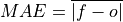The average of the absolute differences between forecasts and verification data. A more robust measure of forecast accuracy than mse which is sensitive to large outlier forecast errors.

Parameters

Notes

 minimum 0.0 maximum ∞ perfect 0.0 orientation negative

References

Jolliffe and Stephenson 

Example

>>> HindcastEnsemble.verify(
...     metric="mae", comparison="e2o", alignment="same_verifs", dim="init"
... )
<xarray.Dataset>
Coordinates:
* lead     (lead) int32 1 2 3 4 5 6 7 8 9 10
skill    <U11 'initialized'
Data variables:
SST      (lead) float64 0.06484 0.06684 0.07407 ... 0.1193 0.1361 0.1462
Attributes:
skill_calculated_by_function:  HindcastEnsemble.verify()
number_of_initializations:     64
number_of_members:             10
alignment:                     same_verifs
metric:                        mae
comparison:                    e2o
dim:                           init
reference:                     []


#### Median Absolute Error#

# Enter any of the below keywords in metric=... for the compute functions.
In : print(f"Keywords: {metric_aliases['median_absolute_error']}")
Keywords: ['median_absolute_error']

climpred.metrics._median_absolute_error(forecast: xarray.Dataset, verif: xarray.Dataset, dim: Optional[Union[str, List[str]]] = None, **metric_kwargs: Any) [source]#

Median Absolute Error.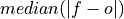The median of the absolute differences between forecasts and verification data. Applying the median function to absolute error makes it more robust to outliers.

Parameters

Notes

 minimum 0.0 maximum ∞ perfect 0.0 orientation negative

Example

>>> HindcastEnsemble.verify(
...     metric="median_absolute_error",
...     comparison="e2o",
...     alignment="same_verifs",
...     dim="init",
... )
<xarray.Dataset>
Coordinates:
* lead     (lead) int32 1 2 3 4 5 6 7 8 9 10
skill    <U11 'initialized'
Data variables:
SST      (lead) float64 0.06077 0.06556 0.06368 ... 0.1131 0.142 0.1466
Attributes:
skill_calculated_by_function:  HindcastEnsemble.verify()
number_of_initializations:     64
number_of_members:             10
alignment:                     same_verifs
metric:                        median_absolute_error
comparison:                    e2o
dim:                           init
reference:                     []


# Enter any of the below keywords in metric=... for the compute functions.

climpred.metrics._spread(forecast: xarray.Dataset, verif: xarray.Dataset, dim: Optional[Union[str, List[str]]] = None, **metric_kwargs: Any) [source]#

Ensemble spread taking the standard deviation over the member dimension.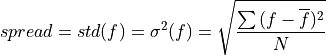Parameters
• forecast – Forecast.

• verif – Verification data (not used).

• dim – Dimension(s) to perform metric over.

• metric_kwargs – see std()

Notes

 minimum 0.0 maximum ∞ perfect obs.std() orientation negative

Example

>>> HindcastEnsemble.verify(
...     comparison="m2o",
...     alignment="same_verifs",
...     dim=["member", "init"],
... )
<xarray.Dataset>
Coordinates:
* lead     (lead) int32 1 2 3 4 5 6 7 8 9 10
skill    <U11 'initialized'
Data variables:
SST      (lead) float64 0.1468 0.1738 0.1922 0.2096 ... 0.2142 0.2178 0.2098
Attributes:
skill_calculated_by_function:  HindcastEnsemble.verify()
number_of_initializations:     64
number_of_members:             10
alignment:                     same_verifs
comparison:                    m2o
dim:                           ['member', 'init']
reference:                     []


#### Multiplicative bias#

# Enter any of the below keywords in metric=... for the compute functions.
In : print(f"Keywords: {metric_aliases['mul_bias']}")
Keywords: ['mul_bias', 'm_b', 'multiplicative_bias']

climpred.metrics._mul_bias(forecast: xarray.Dataset, verif: xarray.Dataset, dim: Optional[Union[str, List[str]]] = None, **metric_kwargs: Any) [source]#

Multiplicative bias.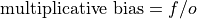Parameters
• forecast – Forecast.

• verif – Verification data.

• dim – Dimension(s) to perform metric over

• metric_kwargs – see xarray.mean

Notes

 minimum -∞ maximum ∞ perfect 1.0 orientation None

Example

>>> HindcastEnsemble.verify(
...     metric="multiplicative_bias",
...     comparison="e2o",
...     alignment="same_verifs",
...     dim="init",
... )
<xarray.Dataset>
Coordinates:
* lead     (lead) int32 1 2 3 4 5 6 7 8 9 10
skill    <U11 'initialized'
Data variables:
SST      (lead) float64 0.719 0.9991 1.072 1.434 ... 1.854 2.128 2.325 2.467
Attributes:
skill_calculated_by_function:  HindcastEnsemble.verify()
number_of_initializations:     64
number_of_members:             10
alignment:                     same_verifs
metric:                        mul_bias
comparison:                    e2o
dim:                           init
reference:                     []


### Normalized Distance Metrics#

Distance metrics like mse can be normalized to 1. The normalization factor depends on the comparison type choosen. For example, the distance between an ensemble member and the ensemble mean is half the distance of an ensemble member with other ensemble members. See _get_norm_factor().

#### Normalized Mean Square Error (NMSE)#

# Enter any of the below keywords in metric=... for the compute functions.
In : print(f"Keywords: {metric_aliases['nmse']}")
Keywords: ['nmse', 'nev']

climpred.metrics._nmse(forecast: xarray.Dataset, verif: xarray.Dataset, dim: Optional[Union[str, List[str]]] = None, **metric_kwargs: Any) [source]#

Compte Normalized MSE (NMSE), also known as Normalized Ensemble Variance (NEV).

Mean Square Error (mse) normalized by the variance of the verification data.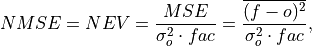where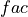is 1 when using comparisons involving the ensemble mean (m2e, e2c, e2o) and 2 when using comparisons involving individual ensemble members (m2c, m2m, m2o). See _get_norm_factor().

Note

climpred uses a single-valued internal reference forecast for the NMSE, in the terminology of Murphy . I.e., we use a single climatological variance of the verification data within the experimental window for normalizing MSE.

Parameters

Notes

 minimum 0.0 maximum ∞ perfect 0.0 orientation negative better than climatology 0.0 - 1.0 worse than climatology > 1.0

References

Griffies and Bryan 

Example

>>> HindcastEnsemble.verify(
...     metric="nmse", comparison="e2o", alignment="same_verifs", dim="init"
... )
<xarray.Dataset>
Coordinates:
* lead     (lead) int32 1 2 3 4 5 6 7 8 9 10
skill    <U11 'initialized'
Data variables:
SST      (lead) float64 0.1732 0.1825 0.217 0.2309 ... 0.5247 0.6749 0.7732
Attributes:
skill_calculated_by_function:  HindcastEnsemble.verify()
number_of_initializations:     64
number_of_members:             10
alignment:                     same_verifs
metric:                        nmse
comparison:                    e2o
dim:                           init
reference:                     []


#### Normalized Mean Absolute Error (NMAE)#

# Enter any of the below keywords in metric=... for the compute functions.
In : print(f"Keywords: {metric_aliases['nmae']}")
Keywords: ['nmae']

climpred.metrics._nmae(forecast: xarray.Dataset, verif: xarray.Dataset, dim: Optional[Union[str, List[str]]] = None, **metric_kwargs: Any) [source]#

Compute Normalized Mean Absolute Error (NMAE).

Mean Absolute Error (mae) normalized by the standard deviation of the verification data.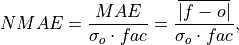whereis 1 when using comparisons involving the ensemble mean (m2e, e2c, e2o) and 2 when using comparisons involving individual ensemble members (m2c, m2m, m2o). See _get_norm_factor().

Note

climpred uses a single-valued internal reference forecast for the NMAE, in the terminology of Murphy . I.e., we use a single climatological standard deviation of the verification data within the experimental window for normalizing MAE.

Parameters

Notes

 minimum 0.0 maximum ∞ perfect 0.0 orientation negative better than climatology 0.0 - 1.0 worse than climatology > 1.0

References

• Murphy 

• Griffies and Bryan 

Example

>>> HindcastEnsemble.verify(
...     metric="nmae", comparison="e2o", alignment="same_verifs", dim="init"
... )
<xarray.Dataset>
Coordinates:
* lead     (lead) int32 1 2 3 4 5 6 7 8 9 10
skill    <U11 'initialized'
Data variables:
SST      (lead) float64 0.3426 0.3532 0.3914 0.3898 ... 0.6303 0.7194 0.7726
Attributes:
skill_calculated_by_function:  HindcastEnsemble.verify()
number_of_initializations:     64
number_of_members:             10
alignment:                     same_verifs
metric:                        nmae
comparison:                    e2o
dim:                           init
reference:                     []


#### Normalized Root Mean Square Error (NRMSE)#

# Enter any of the below keywords in metric=... for the compute functions.
In : print(f"Keywords: {metric_aliases['nrmse']}")
Keywords: ['nrmse']

climpred.metrics._nrmse(forecast: xarray.Dataset, verif: xarray.Dataset, dim: Optional[Union[str, List[str]]] = None, **metric_kwargs: Any) [source]#

Compute Normalized Root Mean Square Error (NRMSE).

Root Mean Square Error (rmse) normalized by the standard deviation of the verification data.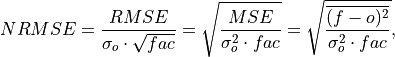whereis 1 when using comparisons involving the ensemble mean (m2e, e2c, e2o) and 2 when using comparisons involving individual ensemble members (m2c, m2m, m2o). See _get_norm_factor().

Note

climpred uses a single-valued internal reference forecast for the NRMSE, in the terminology of Murphy . I.e., we use a single climatological variance of the verification data within the experimental window for normalizing RMSE.

Parameters

Notes

 minimum 0.0 maximum ∞ perfect 0.0 orientation negative better than climatology 0.0 - 1.0 worse than climatology > 1.0

References

Example

>>> HindcastEnsemble.verify(
...     metric="nrmse", comparison="e2o", alignment="same_verifs", dim="init"
... )
<xarray.Dataset>
Coordinates:
* lead     (lead) int32 1 2 3 4 5 6 7 8 9 10
skill    <U11 'initialized'
Data variables:
SST      (lead) float64 0.4161 0.4272 0.4658 0.4806 ... 0.7244 0.8215 0.8793
Attributes:
skill_calculated_by_function:  HindcastEnsemble.verify()
number_of_initializations:     64
number_of_members:             10
alignment:                     same_verifs
metric:                        nrmse
comparison:                    e2o
dim:                           init
reference:                     []


#### Mean Square Error Skill Score (MSESS)#

# Enter any of the below keywords in metric=... for the compute functions.
In : print(f"Keywords: {metric_aliases['msess']}")
Keywords: ['msess', 'ppp', 'msss']

climpred.metrics._msess(forecast: xarray.Dataset, verif: xarray.Dataset, dim: Optional[Union[str, List[str]]] = None, **metric_kwargs: Any) [source]#

Mean Squared Error Skill Score (MSESS).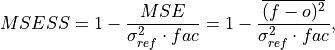whereis 1 when using comparisons involving the ensemble mean (m2e, e2c, e2o) and 2 when using comparisons involving individual ensemble members (m2c, m2m, m2o). See _get_norm_factor().

This skill score can be intepreted as a percentage improvement in accuracy. I.e., it can be multiplied by 100%.

Note

climpred uses a single-valued internal reference forecast for the MSSS, in the terminology of Murphy . I.e., we use a single climatological variance of the verification data within the experimental window for normalizing MSE.

Parameters

Notes

 minimum -∞ maximum 1.0 perfect 1.0 orientation positive better than climatology > 0.0 equal to climatology 0.0 worse than climatology < 0.0

References

• Griffies and Bryan 

• Murphy 

• Pohlmann et al. 

• Bushuk et al. 

Example

>>> HindcastEnsemble.verify(
...     metric="msess", comparison="e2o", alignment="same_verifs", dim="init"
... )
<xarray.Dataset>
Coordinates:
* lead     (lead) int32 1 2 3 4 5 6 7 8 9 10
skill    <U11 'initialized'
Data variables:
SST      (lead) float64 0.8268 0.8175 0.783 0.7691 ... 0.4753 0.3251 0.2268
Attributes:
skill_calculated_by_function:  HindcastEnsemble.verify()
number_of_initializations:     64
number_of_members:             10
alignment:                     same_verifs
metric:                        msess
comparison:                    e2o
dim:                           init
reference:                     []


#### Mean Absolute Percentage Error (MAPE)#

# Enter any of the below keywords in metric=... for the compute functions.
In : print(f"Keywords: {metric_aliases['mape']}")
Keywords: ['mape']

climpred.metrics._mape(forecast: xarray.Dataset, verif: xarray.Dataset, dim: Optional[Union[str, List[str]]] = None, **metric_kwargs: Any) [source]#

Mean Absolute Percentage Error (MAPE).

Mean absolute error (mae) expressed as the fractional error relative to the verification data.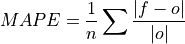Parameters

Notes

 minimum 0.0 maximum ∞ perfect 0.0 orientation negative

Example

>>> HindcastEnsemble.verify(
...     metric="mape", comparison="e2o", alignment="same_verifs", dim="init"
... )
<xarray.Dataset>
Coordinates:
* lead     (lead) int32 1 2 3 4 5 6 7 8 9 10
skill    <U11 'initialized'
Data variables:
SST      (lead) float64 1.536 1.21 1.421 1.149 ... 1.078 1.369 1.833 1.245
Attributes:
skill_calculated_by_function:  HindcastEnsemble.verify()
number_of_initializations:     64
number_of_members:             10
alignment:                     same_verifs
metric:                        mape
comparison:                    e2o
dim:                           init
reference:                     []


#### Symmetric Mean Absolute Percentage Error (sMAPE)#

# Enter any of the below keywords in metric=... for the compute functions.
In : print(f"Keywords: {metric_aliases['smape']}")
Keywords: ['smape']

climpred.metrics._smape(forecast: xarray.Dataset, verif: xarray.Dataset, dim: Optional[Union[str, List[str]]] = None, **metric_kwargs: Any) [source]#

Symmetric Mean Absolute Percentage Error (sMAPE).

Similar to the Mean Absolute Percentage Error (mape), but sums the forecast and observation mean in the denominator.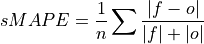Parameters

Notes

 minimum 0.0 maximum 1.0 perfect 0.0 orientation negative

Example

>>> HindcastEnsemble.verify(
...     metric="smape", comparison="e2o", alignment="same_verifs", dim="init"
... )
<xarray.Dataset>
Coordinates:
* lead     (lead) int32 1 2 3 4 5 6 7 8 9 10
skill    <U11 'initialized'
Data variables:
SST      (lead) float64 0.3801 0.3906 0.4044 0.3819 ... 0.4822 0.5054 0.5295
Attributes:
skill_calculated_by_function:  HindcastEnsemble.verify()
number_of_initializations:     64
number_of_members:             10
alignment:                     same_verifs
metric:                        smape
comparison:                    e2o
dim:                           init
reference:                     []


#### Unbiased Anomaly Correlation Coefficient (uACC)#

# Enter any of the below keywords in metric=... for the compute functions.
In : print(f"Keywords: {metric_aliases['uacc']}")
Keywords: ['uacc']

climpred.metrics._uacc(forecast: xarray.Dataset, verif: xarray.Dataset, dim: Optional[Union[str, List[str]]] = None, **metric_kwargs: Any) [source]#

Bushuk’s unbiased Anomaly Correlation Coefficient (uACC).

This is typically used in perfect model studies. Because the perfect model Anomaly Correlation Coefficient (ACC) is strongly state dependent, a standard ACC (e.g. one computed using _pearson_r()) will be highly sensitive to the set of start dates chosen for the perfect model study. The Mean Square Skill Score (MESSS) can be related directly to the ACC as MESSS = ACC^(2) (see Murphy  and Bushuk et al. ), so the unbiased ACC can be derived as uACC = sqrt(MESSS).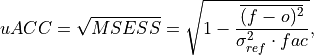whereis 1 when using comparisons involving the ensemble mean (m2e, e2c, e2o) and 2 when using comparisons involving individual ensemble members (m2c, m2m, m2o). See _get_norm_factor().

Note

Because of the square root involved, any negative MSESS values are automatically converted to NaNs.

Parameters
• forecast – Forecast.

• verif – Verification data.

• dim – Dimension(s) to perform metric over.

• comparison – Name comparison needed for normalization factor fac, see _get_norm_factor() (Handled internally by the compute functions)

• metric_kwargs – see xskillscore.mse()

Notes

 minimum 0.0 maximum 1.0 perfect 1.0 orientation positive better than climatology > 0.0 equal to climatology 0.0

References

Example

>>> HindcastEnsemble.verify(
...     metric="uacc", comparison="e2o", alignment="same_verifs", dim="init"
... )
<xarray.Dataset>
Coordinates:
* lead     (lead) int32 1 2 3 4 5 6 7 8 9 10
skill    <U11 'initialized'
Data variables:
SST      (lead) float64 0.9093 0.9041 0.8849 0.877 ... 0.6894 0.5702 0.4763
Attributes:
skill_calculated_by_function:  HindcastEnsemble.verify()
number_of_initializations:     64
number_of_members:             10
alignment:                     same_verifs
metric:                        uacc
comparison:                    e2o
dim:                           init
reference:                     []


### Murphy Decomposition Metrics#

Metrics derived in which decompose the MSESS into a correlation term, a conditional bias term, and an unconditional bias term. See https://www-miklip.dkrz.de/about/murcss/ for a walk through of the decomposition.

#### Standard Ratio#

# Enter any of the below keywords in metric=... for the compute functions.
In : print(f"Keywords: {metric_aliases['std_ratio']}")
Keywords: ['std_ratio']

climpred.metrics._std_ratio(forecast: xarray.Dataset, verif: xarray.Dataset, dim: Optional[Union[str, List[str]]] = None, **metric_kwargs: Any) [source]#

Ratio of standard deviations of the forecast over the verification data.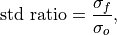whereandare the standard deviations of the forecast and the verification data over the experimental period, respectively.

Parameters
• forecast – Forecast.

• verif – Verification data.

• dim – Dimension(s) to perform metric over.

• metric_kwargs – see xarray.std

Notes

 minimum 0.0 maximum ∞ perfect 1.0 orientation N/A

References

Example

>>> HindcastEnsemble.verify(
...     metric="std_ratio",
...     comparison="e2o",
...     alignment="same_verifs",
...     dim="init",
... )
<xarray.Dataset>
Coordinates:
* lead     (lead) int32 1 2 3 4 5 6 7 8 9 10
skill    <U11 'initialized'
Data variables:
SST      (lead) float64 0.7567 0.8801 0.9726 1.055 ... 1.075 1.094 1.055
Attributes:
skill_calculated_by_function:  HindcastEnsemble.verify()
number_of_initializations:     64
number_of_members:             10
alignment:                     same_verifs
metric:                        std_ratio
comparison:                    e2o
dim:                           init
reference:                     []


#### Conditional Bias#

# Enter any of the below keywords in metric=... for the compute functions.
In : print(f"Keywords: {metric_aliases['conditional_bias']}")
Keywords: ['conditional_bias', 'c_b', 'cond_bias']

climpred.metrics._conditional_bias(forecast: xarray.Dataset, verif: xarray.Dataset, dim: Optional[Union[str, List[str]]] = None, **metric_kwargs: Any) [source]#

Conditional bias between forecast and verification data.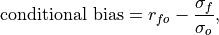whereandare the standard deviations of the forecast and verification data over the experimental period, respectively.

Parameters

Notes

 minimum -∞ maximum 1.0 perfect 0.0 orientation negative

References

Example

>>> HindcastEnsemble.verify(
...     metric="conditional_bias",
...     comparison="e2o",
...     alignment="same_verifs",
...     dim="init",
... )
<xarray.Dataset>
Coordinates:
* lead     (lead) int32 1 2 3 4 5 6 7 8 9 10
skill    <U11 'initialized'
Data variables:
SST      (lead) float64 0.1705 0.03435 -0.05988 ... -0.1436 -0.175 -0.1434
Attributes:
skill_calculated_by_function:  HindcastEnsemble.verify()
number_of_initializations:     64
number_of_members:             10
alignment:                     same_verifs
metric:                        conditional_bias
comparison:                    e2o
dim:                           init
reference:                     []


#### Unconditional Bias#

# Enter any of the below keywords in metric=... for the compute functions.
In : print(f"Keywords: {metric_aliases['unconditional_bias']}")
Keywords: ['unconditional_bias', 'u_b', 'a_b', 'bias', 'additive_bias']


Simple bias of the forecast minus the observations.

climpred.metrics._unconditional_bias(forecast: xarray.Dataset, verif: xarray.Dataset, dim: Optional[Union[str, List[str]]] = None, **metric_kwargs: Any) [source]#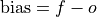Parameters
• forecast – Forecast.

• verif – Verification data.

• dim – Dimension(s) to perform metric over

• metric_kwargs – see xarray.mean

Notes

 minimum -∞ maximum ∞ perfect 0.0 orientation negative

References

Example

>>> HindcastEnsemble.verify(
...     metric="unconditional_bias",
...     comparison="e2o",
...     alignment="same_verifs",
...     dim="init",
... )
<xarray.Dataset>
Coordinates:
* lead     (lead) int32 1 2 3 4 5 6 7 8 9 10
skill    <U11 'initialized'
Data variables:
SST      (lead) float64 -0.01158 -0.02512 -0.0408 ... -0.1322 -0.1445
Attributes:
skill_calculated_by_function:  HindcastEnsemble.verify()
number_of_initializations:     64
number_of_members:             10
alignment:                     same_verifs
metric:                        unconditional_bias
comparison:                    e2o
dim:                           init
reference:                     []


Conditional bias is removed by HindcastEnsemble.remove_bias().

>>> HindcastEnsemble = HindcastEnsemble.remove_bias(alignment="same_verifs")
>>> HindcastEnsemble.verify(
...     metric="unconditional_bias",
...     comparison="e2o",
...     alignment="same_verifs",
...     dim="init",
... )
<xarray.Dataset>
Coordinates:
* lead     (lead) int32 1 2 3 4 5 6 7 8 9 10
skill    <U11 'initialized'
Data variables:
SST      (lead) float64 3.203e-18 -1.068e-18 ... 2.882e-17 -2.776e-17
Attributes:
skill_calculated_by_function:  HindcastEnsemble.verify()
number_of_initializations:     64
number_of_members:             10
alignment:                     same_verifs
metric:                        unconditional_bias
comparison:                    e2o
dim:                           init
reference:                     []


#### Bias Slope#

# Enter any of the below keywords in metric=... for the compute functions.
In : print(f"Keywords: {metric_aliases['bias_slope']}")
Keywords: ['bias_slope']

climpred.metrics._bias_slope(forecast: xarray.Dataset, verif: xarray.Dataset, dim: Optional[Union[str, List[str]]] = None, **metric_kwargs: Any) [source]#

Bias slope between verification data and forecast standard deviations.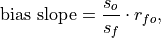where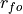is the Pearson product-moment correlation between the forecast and the verification data and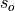and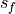are the standard deviations of the verification data and forecast over the experimental period, respectively.

Parameters

Notes

 minimum 0.0 maximum ∞ perfect 1.0 orientation negative

References

Example

>>> HindcastEnsemble.verify(
...     metric="bias_slope",
...     comparison="e2o",
...     alignment="same_verifs",
...     dim="init",
... )
<xarray.Dataset>
Coordinates:
* lead     (lead) int32 1 2 3 4 5 6 7 8 9 10
skill    <U11 'initialized'
Data variables:
SST      (lead) float64 0.7016 0.8049 0.8877 0.9836 ... 1.002 1.004 0.961
Attributes:
skill_calculated_by_function:  HindcastEnsemble.verify()
number_of_initializations:     64
number_of_members:             10
alignment:                     same_verifs
metric:                        bias_slope
comparison:                    e2o
dim:                           init
reference:                     []


#### Murphy’s Mean Square Error Skill Score#

# Enter any of the below keywords in metric=... for the compute functions.
In : print(f"Keywords: {metric_aliases['msess_murphy']}")
Keywords: ['msess_murphy', 'msss_murphy']

climpred.metrics._msess_murphy(forecast: xarray.Dataset, verif: xarray.Dataset, dim: Optional[Union[str, List[str]]] = None, **metric_kwargs: Any) [source]#

Murphy’s Mean Square Error Skill Score (MSESS).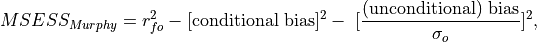where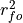represents the Pearson product-moment correlation coefficient between the forecast and verification data andrepresents the standard deviation of the verification data over the experimental period. See conditional_bias and unconditional_bias for their respective formulations.

Parameters

Notes

 minimum -∞ maximum 1.0 perfect 1.0 orientation positive

References

Example

>>> HindcastEnsemble = HindcastEnsemble.remove_bias(alignment="same_verifs")
>>> HindcastEnsemble.verify(
...     metric="msess_murphy",
...     comparison="e2o",
...     dim="init",
...     alignment="same_verifs",
... )
<xarray.Dataset>
Coordinates:
* lead     (lead) int32 1 2 3 4 5 6 7 8 9 10
skill    <U11 'initialized'
Data variables:
SST      (lead) float64 0.8306 0.8351 0.8295 0.8532 ... 0.8471 0.813 0.8097
Attributes:
skill_calculated_by_function:  HindcastEnsemble.verify()
number_of_initializations:     64
number_of_members:             10
alignment:                     same_verifs
metric:                        msess_murphy
comparison:                    e2o
dim:                           init
reference:                     []


## Probabilistic#

Probabilistic metrics include the spread of the ensemble simulations in their calculations and assign a probability value between 0 and 1 to their forecasts .

### Continuous Ranked Probability Score (CRPS)#

# Enter any of the below keywords in metric=... for the compute functions.
In : print(f"Keywords: {metric_aliases['crps']}")
Keywords: ['crps']

climpred.metrics._crps(forecast: xarray.Dataset, verif: xarray.Dataset, dim: Optional[Union[str, List[str]]] = None, **metric_kwargs: Any) [source]#

Continuous Ranked Probability Score (CRPS).

The CRPS can also be considered as the probabilistic Mean Absolute Error (mae). It compares the empirical distribution of an ensemble forecast to a scalar observation. Smaller scores indicate better skill.where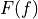is the cumulative distribution function (CDF) of the forecast (since the verification data are not assigned a probability), and H() is the Heaviside step function where the value is 1 if the argument is positive (i.e., the forecast overestimates verification data) or zero (i.e., the forecast equals verification data) and is 0 otherwise (i.e., the forecast is less than verification data).

Note

The CRPS is expressed in the same unit as the observed variable. It generalizes the Mean Absolute Error (MAE), and reduces to the MAE if the forecast is determinstic.

Parameters
• forecast – Forecast with member dim.

• verif – Verification data without member dim.

• dim – Dimension to apply metric over. Expects at least member. Other dimensions are passed to xskillscore and averaged.

• metric_kwargs – optional, see xskillscore.crps_ensemble()

Notes

 minimum 0.0 maximum ∞ perfect 0.0 orientation negative

References

Example

>>> HindcastEnsemble.verify(
...     metric="crps", comparison="m2o", dim="member", alignment="same_inits"
... )
<xarray.Dataset>
Coordinates:
* init        (init) object 1954-01-01 00:00:00 ... 2005-01-01 00:00:00
* lead        (lead) int32 1 2 3 4 5 6 7 8 9 10
valid_time  (lead, init) object 1955-01-01 00:00:00 ... 2015-01-01 00:00:00
skill       <U11 'initialized'
Data variables:
SST         (lead, init) float64 0.1722 0.1202 0.01764 ... 0.05428 0.1638
Attributes:
skill_calculated_by_function:  HindcastEnsemble.verify()
number_of_members:             10
alignment:                     same_inits
metric:                        crps
comparison:                    m2o
dim:                           member
reference:                     []


### Continuous Ranked Probability Skill Score (CRPSS)#

# Enter any of the below keywords in metric=... for the compute functions.
In : print(f"Keywords: {metric_aliases['crpss']}")
Keywords: ['crpss']

climpred.metrics._crpss(forecast: xarray.Dataset, verif: xarray.Dataset, dim: Optional[Union[str, List[str]]] = None, **metric_kwargs: Any) [source]#

Continuous Ranked Probability Skill Score.

This can be used to assess whether the ensemble spread is a useful measure for the forecast uncertainty by comparing the CRPS of the ensemble forecast to that of a reference forecast with the desired spread.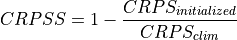Note

When assuming a Gaussian distribution of forecasts, use default gaussian=True. If not gaussian, you may specify the distribution type, xmin/xmax/tolerance for integration (see xskillscore.crps_quadrature()).

Parameters

Notes

 minimum -∞ maximum 1.0 perfect 1.0 orientation positive better than climatology > 0.0 worse than climatology < 0.0

References

• Matheson and Winkler 

• Gneiting and Raftery 

Example

>>> HindcastEnsemble.verify(
...     metric="crpss", comparison="m2o", alignment="same_inits", dim="member"
... )
<xarray.Dataset>
Coordinates:
* init        (init) object 1954-01-01 00:00:00 ... 2005-01-01 00:00:00
* lead        (lead) int32 1 2 3 4 5 6 7 8 9 10
valid_time  (lead, init) object 1955-01-01 00:00:00 ... 2015-01-01 00:00:00
skill       <U11 'initialized'
Data variables:
SST         (lead, init) float64 0.2644 0.3636 0.7376 ... 0.7702 0.5126
Attributes:
skill_calculated_by_function:  HindcastEnsemble.verify()
number_of_members:             10
alignment:                     same_inits
metric:                        crpss
comparison:                    m2o
dim:                           member
reference:                     []

>>> import scipy
...     metric="crpss",
...     comparison="m2m",
...     dim="member",
...     gaussian=False,
...     cdf_or_dist=scipy.stats.norm,
...     xmin=-10,
...     xmax=10,
...     tol=1e-6,
... )
<xarray.Dataset>
Dimensions:  (init: 12, lead: 2, member: 9)
Coordinates:
* init     (init) object 3014-01-01 00:00:00 ... 3257-01-01 00:00:00
* member   (member) int64 1 2 3 4 5 6 7 8 9
Data variables:
tos      (lead, init, member) float64 0.9931 0.9932 0.9932 ... 0.9947 0.9947


### Continuous Ranked Probability Skill Score Ensemble Spread#

# Enter any of the below keywords in metric=... for the compute functions.
In : print(f"Keywords: {metric_aliases['crpss_es']}")
Keywords: ['crpss_es']

climpred.metrics._crpss_es(forecast: xarray.Dataset, verif: xarray.Dataset, dim: Optional[Union[str, List[str]]] = None, **metric_kwargs: Any) [source]#

Continuous Ranked Probability Skill Score Ensemble Spread.

If the ensemble variance is smaller than the observed mse, the ensemble is said to be under-dispersive (or overconfident). An ensemble with variance larger than the verification data indicates one that is over-dispersive (underconfident).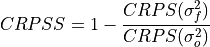Parameters
• forecast – Forecast with member dim.

• verif – Verification data without member dim.

• dim – Dimension to apply metric over. Expects at least member. Other dimensions are passed to xskillscore and averaged.

• metric_kwargs – see xskillscore.crps_ensemble() and xskillscore.mse()

Notes

 minimum -∞ maximum 0.0 perfect 0.0 orientation positive under-dispersive > 0.0 over-dispersive < 0.0

References

Example

>>> HindcastEnsemble.verify(
...     metric="crpss_es",
...     comparison="m2o",
...     alignment="same_verifs",
...     dim="member",
... )
<xarray.Dataset>
Coordinates:
* init        (init) object 1964-01-01 00:00:00 ... 2015-01-01 00:00:00
* lead        (lead) int32 1 2 3 4 5 6 7 8 9 10
valid_time  (init) object 1964-01-01 00:00:00 ... 2015-01-01 00:00:00
skill       <U11 'initialized'
Data variables:
SST         (lead, init) float64 -0.01121 -0.05575 ... -0.1263 -0.007483
Attributes:
skill_calculated_by_function:  HindcastEnsemble.verify()
number_of_members:             10
alignment:                     same_verifs
metric:                        crpss_es
comparison:                    m2o
dim:                           member
reference:                     []


### Brier Score#

# Enter any of the below keywords in metric=... for the compute functions.
In : print(f"Keywords: {metric_aliases['brier_score']}")
Keywords: ['brier_score', 'brier', 'bs']

climpred.metrics._brier_score(forecast: xarray.Dataset, verif: xarray.Dataset, dim: Optional[Union[str, List[str]]] = None, **metric_kwargs: Any) [source]#

Brier Score for binary events.

The Mean Square Error (mse) of probabilistic two-category forecasts where the verification data are either 0 (no occurrence) or 1 (occurrence) and forecast probability may be arbitrarily distributed between occurrence and non-occurrence. The Brier Score equals zero for perfect (single-valued) forecasts and one for forecasts that are always incorrect.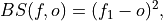where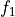is the forecast probability of.

Note

The Brier Score requires that the observation is binary, i.e., can be described as one (a “hit”) or zero (a “miss”). So either provide a function with with binary outcomes logical in metric_kwargs or create binary verifs and probability forecasts by hindcast.map(logical).mean(“member”). This Brier Score is not the original formula given in Brier .

Parameters
• forecast – Raw forecasts with member dimension if logical provided in metric_kwargs. Probability forecasts in [0, 1] if logical is not provided.

• verif – Verification data without member dim. Raw verification if logical provided, else binary verification.

• dim – Dimensions to aggregate. Requires member if logical provided in metric_kwargsto create probability forecasts. If logical not provided in metric_kwargs, should not include member.

• logical (callable) – Function with bool result to be applied to verification data and forecasts and then mean("member") to get forecasts and verification data in interval [0, 1]. see xskillscore.brier_score()

Notes

 minimum 0.0 maximum 1.0 perfect 0.0 orientation negative

References

Example

Define a boolean/logical: Function for binary scoring:

>>> def pos(x):
...     return x > 0  # checking binary outcomes
...


Option 1. Pass with keyword logical: (specifically designed for PerfectModelEnsemble, where binary verification can only be created after comparison)

>>> HindcastEnsemble.verify(
...     metric="brier_score",
...     comparison="m2o",
...     dim=["member", "init"],
...     alignment="same_verifs",
...     logical=pos,
... )
<xarray.Dataset>
Coordinates:
* lead     (lead) int32 1 2 3 4 5 6 7 8 9 10
skill    <U11 'initialized'
Data variables:
SST      (lead) float64 0.115 0.1121 0.1363 0.125 ... 0.1654 0.1675 0.1873
Attributes:
skill_calculated_by_function:  HindcastEnsemble.verify()
number_of_initializations:     64
number_of_members:             10
alignment:                     same_verifs
metric:                        brier_score
comparison:                    m2o
dim:                           ['member', 'init']
reference:                     []
logical:                       Callable


Option 2. Pre-process to generate a binary multi-member forecast and binary verification product:

>>> HindcastEnsemble.map(pos).verify(
...     metric="brier_score",
...     comparison="m2o",
...     dim=["member", "init"],
...     alignment="same_verifs",
... )
<xarray.Dataset>
Coordinates:
* lead     (lead) int32 1 2 3 4 5 6 7 8 9 10
skill    <U11 'initialized'
Data variables:
SST      (lead) float64 0.115 0.1121 0.1363 0.125 ... 0.1654 0.1675 0.1873
Attributes:
skill_calculated_by_function:  HindcastEnsemble.verify()
number_of_initializations:     64
number_of_members:             10
alignment:                     same_verifs
metric:                        brier_score
comparison:                    m2o
dim:                           ['member', 'init']
reference:                     []


Option 3. Pre-process to generate a probability forecast and binary verification product. because member not present in hindcast anymore, use comparison="e2o" and dim="init":

>>> HindcastEnsemble.map(pos).mean("member").verify(
...     metric="brier_score",
...     comparison="e2o",
...     dim="init",
...     alignment="same_verifs",
... )
<xarray.Dataset>
Coordinates:
* lead     (lead) int32 1 2 3 4 5 6 7 8 9 10
skill    <U11 'initialized'
Data variables:
SST      (lead) float64 0.115 0.1121 0.1363 0.125 ... 0.1654 0.1675 0.1873
Attributes:
skill_calculated_by_function:  HindcastEnsemble.verify()
number_of_initializations:     64
alignment:                     same_verifs
metric:                        brier_score
comparison:                    e2o
dim:                           init
reference:                     []


### Threshold Brier Score#

# Enter any of the below keywords in metric=... for the compute functions.
In : print(f"Keywords: {metric_aliases['threshold_brier_score']}")
Keywords: ['threshold_brier_score', 'tbs']

climpred.metrics._threshold_brier_score(forecast: xarray.Dataset, verif: xarray.Dataset, dim: Optional[Union[str, List[str]]] = None, **metric_kwargs: Any) [source]#

Brier score of an ensemble for exceeding given thresholds.where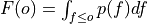is the cumulative distribution function (CDF) of the forecast distribution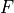,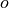is a point estimate of the true observation (observational error is neglected),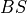denotes the Brier score and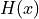denotes the Heaviside step function, which we define here as equal to 1 for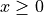and 0 otherwise.

Parameters
• forecast – Forecast with member dim.

• verif – Verification data without member dim.

• dim – Dimension to apply metric over. Expects at least member. Other dimensions are passed to xskillscore and averaged.

• threshold (int, float, xarray.Dataset, xr.DataArray) – Threshold to check exceedance, see xskillscore.threshold_brier_score().

• metric_kwargs – optional, see xskillscore.threshold_brier_score()

Notes

 minimum 0.0 maximum 1.0 perfect 0.0 orientation negative

References

Example

>>> # get threshold brier score for each init
>>> HindcastEnsemble.verify(
...     metric="threshold_brier_score",
...     comparison="m2o",
...     dim="member",
...     threshold=0.2,
...     alignment="same_inits",
... )
<xarray.Dataset>
Coordinates:
* init        (init) object 1954-01-01 00:00:00 ... 2005-01-01 00:00:00
* lead        (lead) int32 1 2 3 4 5 6 7 8 9 10
valid_time  (lead, init) object 1955-01-01 00:00:00 ... 2015-01-01 00:00:00
threshold   float64 0.2
skill       <U11 'initialized'
Data variables:
SST         (lead, init) float64 0.0 0.0 0.0 0.0 0.0 ... 0.25 0.36 0.09 0.01
Attributes:
skill_calculated_by_function:  HindcastEnsemble.verify()
number_of_members:             10
alignment:                     same_inits
metric:                        threshold_brier_score
comparison:                    m2o
dim:                           member
reference:                     []
threshold:                     0.2

>>> # multiple thresholds averaging over init dimension
>>> HindcastEnsemble.verify(
...     metric="threshold_brier_score",
...     comparison="m2o",
...     dim=["member", "init"],
...     threshold=[0.2, 0.3],
...     alignment="same_verifs",
... )
<xarray.Dataset>
Coordinates:
* lead       (lead) int32 1 2 3 4 5 6 7 8 9 10
* threshold  (threshold) float64 0.2 0.3
skill      <U11 'initialized'
Data variables:
SST        (lead, threshold) float64 0.08712 0.005769 ... 0.1312 0.01923
Attributes:
skill_calculated_by_function:  HindcastEnsemble.verify()
number_of_initializations:     64
number_of_members:             10
alignment:                     same_verifs
metric:                        threshold_brier_score
comparison:                    m2o
dim:                           ['member', 'init']
reference:                     []
threshold:                     [0.2, 0.3]


### Ranked Probability Score#

# Enter any of the below keywords in metric=... for the compute functions.
In : print(f"Keywords: {metric_aliases['rps']}")
Keywords: ['rps']

climpred.metrics._rps(forecast: xarray.Dataset, verif: xarray.Dataset, dim: Optional[Union[str, List[str]]] = None, **metric_kwargs: Any) [source]#

Ranked Probability Score.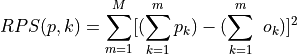Parameters
• forecast – Forecasts.

• verif – Verification.

• dim – Dimensions to aggregate.

• **metric_kwargs, see :py:func:.xskillscore.rps

Note

If category_edges is xr.Dataset or tuple of xr.Datasets, climpred will broadcast the grouped dimensions season, month, weekofyear, dayfofyear onto the dimensions init for forecast and time for observations. see climpred.utils.broadcast_time_grouped_to_time.

Notes

 minimum 0.0 maximum ∞ perfect 0.0 orientation negative

Example

>>> category_edges = np.array([-0.5, 0.0, 0.5, 1.0])
>>> HindcastEnsemble.verify(
...     metric="rps",
...     comparison="m2o",
...     dim=["member", "init"],
...     alignment="same_verifs",
...     category_edges=category_edges,
... )
<xarray.Dataset>
Coordinates:
* lead                        (lead) int32 1 2 3 4 5 6 7 8 9 10
observations_category_edge  <U67 '[-np.inf, -0.5), [-0.5, 0.0), [0.0, 0.5...
forecasts_category_edge     <U67 '[-np.inf, -0.5), [-0.5, 0.0), [0.0, 0.5...
skill                       <U11 'initialized'
Data variables:
SST                         (lead) float64 0.115 0.1123 ... 0.1687 0.1875
Attributes:
skill_calculated_by_function:  HindcastEnsemble.verify()
number_of_initializations:     64
number_of_members:             10
alignment:                     same_verifs
metric:                        rps
comparison:                    m2o
dim:                           ['member', 'init']
reference:                     []
category_edges:                [-0.5  0.   0.5  1. ]


Provide category_edges as xarray.Dataset for category edges varying along dimensions.

>>> category_edges = (
...     xr.DataArray([9.5, 10.0, 10.5, 11.0], dims="category_edge")
...     .assign_coords(category_edge=[9.5, 10.0, 10.5, 11.0])
...     .to_dataset(name="tos")
... )
>>> # category_edges = np.array([9.5, 10., 10.5, 11.]) # identical
>>> PerfectModelEnsemble.verify(
...     metric="rps",
...     comparison="m2c",
...     dim=["member", "init"],
...     category_edges=category_edges,
... )
<xarray.Dataset>
Coordinates:
* lead                        (lead) int64 1 2 3 4 5 6 7 ... 15 16 17 18 19 20
observations_category_edge  <U71 '[-np.inf, 9.5), [9.5, 10.0), [10.0, 10....
forecasts_category_edge     <U71 '[-np.inf, 9.5), [9.5, 10.0), [10.0, 10....
Data variables:
tos                         (lead) float64 0.08951 0.1615 ... 0.1399 0.2274
Attributes:
skill_calculated_by_function:  PerfectModelEnsemble.verify()
number_of_initializations:     12
number_of_members:             10
metric:                        rps
comparison:                    m2c
dim:                           ['member', 'init']
reference:                     []
category_edges:                <xarray.Dataset>\nDimensions:        (cate...


Provide category_edges as tuple for different category edges to categorize forecasts and observations.

>>> q = [1 / 3, 2 / 3]  # terciles by month
>>> forecast_edges = (
...     HindcastEnsemble.get_initialized()
...     .groupby("init.month")
...     .quantile(q=q, dim=["init", "member"])
...     .rename({"quantile": "category_edge"})
... )
>>> obs_edges = (
...     HindcastEnsemble.get_observations()
...     .groupby("time.month")
...     .quantile(q=q, dim="time")
...     .rename({"quantile": "category_edge"})
... )
>>> category_edges = (obs_edges, forecast_edges)
>>> HindcastEnsemble.verify(
...     metric="rps",
...     comparison="m2o",
...     dim=["member", "init"],
...     alignment="same_verifs",
...     category_edges=category_edges,
... )
<xarray.Dataset>
Coordinates:
* lead                        (lead) int32 1 2 3 4 5 6 7 8 9 10
observations_category_edge  <U101 '[-np.inf, 0.3333333333333333), [0.3333...
forecasts_category_edge     <U101 '[-np.inf, 0.3333333333333333), [0.3333...
skill                       <U11 'initialized'
Data variables:
SST                         (lead) float64 0.1248 0.1756 ... 0.3081 0.3413
Attributes:
skill_calculated_by_function:  HindcastEnsemble.verify()
number_of_initializations:     64
number_of_members:             10
alignment:                     same_verifs
metric:                        rps
comparison:                    m2o
dim:                           ['member', 'init']
reference:                     []
category_edges:                (<xarray.Dataset>\nDimensions:        (mon...


### Reliability#

# Enter any of the below keywords in metric=... for the compute functions.
In : print(f"Keywords: {metric_aliases['reliability']}")
Keywords: ['reliability']

climpred.metrics._reliability(forecast: xarray.Dataset, verif: xarray.Dataset, dim: Optional[Union[str, List[str]]] = None, **metric_kwargs: Any) [source]#

Reliability.

Returns the data required to construct the reliability diagram for an event. The the relative frequencies of occurrence of an event for a range of forecast probability bins.

Parameters
• forecast – Raw forecasts with member dimension if logical provided in metric_kwargs. Probability forecasts in [0, 1] if logical is not provided.

• verif – Verification data without member dim. Raw verification if logical provided, else binary verification.

• dim – Dimensions to aggregate. Requires member if logical provided in metric_kwargsto create probability forecasts. If logical not provided in metric_kwargs, should not include member.

• logical – Function with bool result to be applied to verification data and forecasts and then mean("member") to get forecasts and verification data in interval [0, 1]. Passed via metric_kwargs.

• probability_bin_edges (array_like, optional) – Probability bin edges used to compute the reliability. Bins include the left most edge, but not the right. Passed via metric_kwargs. Defaults to 6 equally spaced edges between 0 and 1+1e-8.

Returns

reliability – The relative frequency of occurrence for each probability bin

Notes

 perfect flat distribution

Example

Define a boolean/logical: Function for binary scoring:

>>> def pos(x):
...     return x > 0  # checking binary outcomes
...


Option 1. Pass with keyword logical: (especially designed for PerfectModelEnsemble, where binary verification can only be created after comparison))

>>> HindcastEnsemble.verify(
...     metric="reliability",
...     comparison="m2o",
...     dim=["member", "init"],
...     alignment="same_verifs",
...     logical=pos,
... )
<xarray.Dataset>
Coordinates:
* lead                  (lead) int32 1 2 3 4 5 6 7 8 9 10
* forecast_probability  (forecast_probability) float64 0.1 0.3 0.5 0.7 0.9
SST_samples           (lead, forecast_probability) float64 22.0 5.0 ... 13.0
skill                 <U11 'initialized'
Data variables:
SST                   (lead, forecast_probability) float64 0.09091 ... 1.0
Attributes:
skill_calculated_by_function:  HindcastEnsemble.verify()
number_of_initializations:     64
number_of_members:             10
alignment:                     same_verifs
metric:                        reliability
comparison:                    m2o
dim:                           ['member', 'init']
reference:                     []
logical:                       Callable


Option 2. Pre-process to generate a binary forecast and verification product:

>>> HindcastEnsemble.map(pos).verify(
...     metric="reliability",
...     comparison="m2o",
...     dim=["init", "member"],
...     alignment="same_verifs",
... )
<xarray.Dataset>
Coordinates:
* lead                  (lead) int32 1 2 3 4 5 6 7 8 9 10
* forecast_probability  (forecast_probability) float64 0.1 0.3 0.5 0.7 0.9
SST_samples           (lead, forecast_probability) float64 22.0 5.0 ... 13.0
skill                 <U11 'initialized'
Data variables:
SST                   (lead, forecast_probability) float64 0.09091 ... 1.0
Attributes:
skill_calculated_by_function:  HindcastEnsemble.verify()
number_of_initializations:     64
number_of_members:             10
alignment:                     same_verifs
metric:                        reliability
comparison:                    m2o
dim:                           ['init', 'member']
reference:                     []


Option 3. Pre-process to generate a probability forecast and binary verification product. because member not present in hindcast, use comparison="e2o" and dim="init":

>>> HindcastEnsemble.map(pos).mean("member").verify(
...     metric="reliability",
...     comparison="e2o",
...     dim="init",
...     alignment="same_verifs",
... )
<xarray.Dataset>
Coordinates:
* lead                  (lead) int32 1 2 3 4 5 6 7 8 9 10
* forecast_probability  (forecast_probability) float64 0.1 0.3 0.5 0.7 0.9
SST_samples           (lead, forecast_probability) float64 22.0 5.0 ... 13.0
skill                 <U11 'initialized'
Data variables:
SST                   (lead, forecast_probability) float64 0.09091 ... 1.0
Attributes:
skill_calculated_by_function:  HindcastEnsemble.verify()
number_of_initializations:     64
alignment:                     same_verifs
metric:                        reliability
comparison:                    e2o
dim:                           init
reference:                     []


### Discrimination#

# Enter any of the below keywords in metric=... for the compute functions.
In : print(f"Keywords: {metric_aliases['discrimination']}")
Keywords: ['discrimination']

climpred.metrics._discrimination(forecast: xarray.Dataset, verif: xarray.Dataset, dim: Optional[Union[str, List[str]]] = None, **metric_kwargs: Any) [source]#

Discrimination.

Return the data required to construct the discrimination diagram for an event. The histogram of forecasts likelihood when observations indicate an event has occurred and has not occurred.

Parameters
• forecast – Raw forecasts with member dimension if logical provided in metric_kwargs. Probability forecasts in [0, 1] if logical is not provided.

• verif – Verification data without member dim. Raw verification if logical provided, else binary verification.

• dim – Dimensions to aggregate. Requires member if logical provided in metric_kwargs to create probability forecasts. If logical not provided in metric_kwargs, should not include member. At least one dimension other than memberis required.

• logical – Function with bool result to be applied to verification data and forecasts and then mean("member") to get forecasts and verification data in interval [0, 1]. Passed via metric_kwargs.

• probability_bin_edges (array_like, optional) – Probability bin edges used to compute the histograms. Bins include the left most edge, but not the right. Passed via metric_kwargs. Defaults to 6 equally spaced edges between 0 and 1+1e-8.

Returns

Discrimination with added dimension event containing the histograms of forecast probabilities when the event was observed and not observed.

Notes

 perfect distinct distributions

Example

Define a boolean/logical: Function for binary scoring:

>>> def pos(x):
...     return x > 0  # checking binary outcomes
...


Option 1. Pass with keyword logical: (especially designed for PerfectModelEnsemble, where binary verification can only be created after comparison)

>>> HindcastEnsemble.verify(
...     metric="discrimination",
...     comparison="m2o",
...     dim=["member", "init"],
...     alignment="same_verifs",
...     logical=pos,
... )
<xarray.Dataset>
Dimensions:               (forecast_probability: 5, lead: 10, event: 2)
Coordinates:
* forecast_probability  (forecast_probability) float64 0.1 0.3 0.5 0.7 0.9
* event                 (event) bool True False
* lead                  (lead) int32 1 2 3 4 5 6 7 8 9 10
skill                 <U11 'initialized'
Data variables:
SST                   (lead, event, forecast_probability) float64 0.07407...
Attributes:
skill_calculated_by_function:  HindcastEnsemble.verify()
number_of_initializations:     64
number_of_members:             10
alignment:                     same_verifs
metric:                        discrimination
comparison:                    m2o
dim:                           ['member', 'init']
reference:                     []
logical:                       Callable


Option 2. Pre-process to generate a binary forecast and verification product:

>>> HindcastEnsemble.map(pos).verify(
...     metric="discrimination",
...     comparison="m2o",
...     dim=["member", "init"],
...     alignment="same_verifs",
... )
<xarray.Dataset>
Dimensions:               (forecast_probability: 5, lead: 10, event: 2)
Coordinates:
* forecast_probability  (forecast_probability) float64 0.1 0.3 0.5 0.7 0.9
* event                 (event) bool True False
* lead                  (lead) int32 1 2 3 4 5 6 7 8 9 10
skill                 <U11 'initialized'
Data variables:
SST                   (lead, event, forecast_probability) float64 0.07407...
Attributes:
skill_calculated_by_function:  HindcastEnsemble.verify()
number_of_initializations:     64
number_of_members:             10
alignment:                     same_verifs
metric:                        discrimination
comparison:                    m2o
dim:                           ['member', 'init']
reference:                     []


Option 3. Pre-process to generate a probability forecast and binary verification product. because member not present in hindcast, use comparison="e2o" and dim="init":

>>> HindcastEnsemble.map(pos).mean("member").verify(
...     metric="discrimination",
...     comparison="e2o",
...     dim="init",
...     alignment="same_verifs",
... )
<xarray.Dataset>
Dimensions:               (forecast_probability: 5, lead: 10, event: 2)
Coordinates:
* forecast_probability  (forecast_probability) float64 0.1 0.3 0.5 0.7 0.9
* event                 (event) bool True False
* lead                  (lead) int32 1 2 3 4 5 6 7 8 9 10
skill                 <U11 'initialized'
Data variables:
SST                   (lead, event, forecast_probability) float64 0.07407...
Attributes:
skill_calculated_by_function:  HindcastEnsemble.verify()
number_of_initializations:     64
alignment:                     same_verifs
metric:                        discrimination
comparison:                    e2o
dim:                           init
reference:                     []


### Rank Histogram#

# Enter any of the below keywords in metric=... for the compute functions.
In : print(f"Keywords: {metric_aliases['rank_histogram']}")
Keywords: ['rank_histogram']

climpred.metrics._rank_histogram(forecast: xarray.Dataset, verif: xarray.Dataset, dim: Optional[Union[str, List[str]]] = None, **metric_kwargs: Any) [source]#

Rank histogram or Talagrand diagram.

Parameters
• forecast – Raw forecasts with member dimension.

• verif – Verification data without member dim.

• dim – Dimensions to aggregate. Requires to contain member and at least one additional dimension.

Notes

 flat perfect slope biased u-shaped overconfident/underdisperive dome-shaped underconfident/overdisperive

Example

>>> HindcastEnsemble.verify(
...     metric="rank_histogram",
...     comparison="m2o",
...     dim=["member", "init"],
...     alignment="same_verifs",
... )
<xarray.Dataset>
Coordinates:
* rank     (rank) float64 1.0 2.0 3.0 4.0 5.0 6.0 7.0 8.0 9.0 10.0 11.0
* lead     (lead) int32 1 2 3 4 5 6 7 8 9 10
skill    <U11 'initialized'
Data variables:
SST      (lead, rank) int64 12 3 2 1 1 3 1 2 6 5 16 ... 0 1 0 0 3 0 2 6 6 34
Attributes:
skill_calculated_by_function:  HindcastEnsemble.verify()
number_of_initializations:     64
number_of_members:             10
alignment:                     same_verifs
metric:                        rank_histogram
comparison:                    m2o
dim:                           ['member', 'init']
reference:                     []

>>> PerfectModelEnsemble.verify(
...     metric="rank_histogram", comparison="m2c", dim=["member", "init"]
... )
<xarray.Dataset>
Coordinates:
* lead     (lead) int64 1 2 3 4 5 6 7 8 9 10 11 12 13 14 15 16 17 18 19 20
* rank     (rank) float64 1.0 2.0 3.0 4.0 5.0 6.0 7.0 8.0 9.0 10.0
Data variables:
tos      (lead, rank) int64 1 4 2 1 2 1 0 0 0 1 2 ... 0 2 0 1 2 1 0 3 1 2 0
Attributes:
skill_calculated_by_function:  PerfectModelEnsemble.verify()
number_of_initializations:     12
number_of_members:             10
metric:                        rank_histogram
comparison:                    m2c
dim:                           ['member', 'init']
reference:                     []


# Enter any of the below keywords in metric=... for the compute functions.
In : print(f"Keywords: {metric_aliases['less']}")
Keywords: ['less']

climpred.metrics._less(forecast: xarray.Dataset, verif: xarray.Dataset, dim: Optional[Union[str, List[str]]] = None, **metric_kwargs: Any) [source]#Parameters
• forecast – Forecasts.

• verif – Verification.

• dim – The dimension(s) over which to aggregate. Defaults to None, meaning aggregation over all dims other than lead.

Returns

less – reduced by dimensions dim

Notes

 maximum ∞ positive overdisperive / underconfident perfect 0 negative underdisperive / overconfident minimum -∞ orientation None

Example

>>> # better detrend before
>>> from climpred.stats import rm_poly
>>> HindcastEnsemble.map(rm_poly, dim="init_or_time", deg=2).verify(
...     metric="less",
...     comparison="m2o",
...     dim=["member", "init"],
...     alignment="same_verifs",
... ).SST
array([ 0.12633664, -0.12707636, -0.26143181, -0.25096537, -0.29267366,
-0.2905725 , -0.43579508, -0.33774947, -0.46008438, -0.61010386])
Coordinates:
* lead     (lead) int32 1 2 3 4 5 6 7 8 9 10
skill    <U11 'initialized'
Attributes:
units:    None


References

## Contingency-based metrics#

### Contingency#

A number of metrics can be derived from a contingency table. To use this in climpred, run .verify(metric='contingency', score=...) where score can be chosen from xskillscore.

climpred.metrics._contingency(forecast, verif, score='table', dim=None, **metric_kwargs)[source]#

Contingency table.

Parameters
• forecast – Raw forecasts.

• verif – Verification data.

• dim – Dimensions to aggregate.

• score (str) – Score derived from contingency table. Attribute from Contingency. Use score=table to return a contingency table or any other contingency score, e.g. score=hit_rate.

• observation_category_edges (array_like) – Category bin edges used to compute the observations CDFs. Bins include the left most edge, but not the right. Passed via metric_kwargs.

• forecast_category_edges (array_like) – Category bin edges used to compute the forecast CDFs. Bins include the left most edge, but not the right. Passed via metric_kwargs

Example

>>> category_edges = np.array([-0.5, 0.0, 0.5, 1.0])
>>> HindcastEnsemble.verify(
...     metric="contingency",
...     score="table",
...     comparison="m2o",
...     dim=["member", "init"],
...     alignment="same_verifs",
...     observation_category_edges=category_edges,
...     forecast_category_edges=category_edges,
<xarray.DataArray 'SST' (lead: 2, observations_category: 3,
forecasts_category: 3)>
array([[[221,  29,   0],
[ 53, 217,   0],
[  0,   0,   0]],

[[234,  16,   0],
[ 75, 194,   1],
[  0,   0,   0]]])
Coordinates:
observations_category_bounds  (observations_category) <U11 '[-0.5, 0.0)' ...
forecasts_category_bounds     (forecasts_category) <U11 '[-0.5, 0.0)' ......
* observations_category         (observations_category) int64 1 2 3
* forecasts_category            (forecasts_category) int64 1 2 3
skill                         <U11 'initialized'
Attributes:
units:    None

>>> # contingency-based dichotomous accuracy score
>>> category_edges = np.array([9.5, 10.0, 10.5])
>>> PerfectModelEnsemble.verify(
...     metric="contingency",
...     score="hit_rate",
...     comparison="m2c",
...     dim=["member", "init"],
...     observation_category_edges=category_edges,
...     forecast_category_edges=category_edges,
... )
<xarray.Dataset>
Coordinates:
* lead     (lead) int64 1 2 3 4 5 6 7 8 9 10 11 12 13 14 15 16 17 18 19 20
Data variables:
tos      (lead) float64 1.0 1.0 1.0 1.0 0.9091 ... 1.0 1.0 1.0 nan 1.0
Attributes:
skill_calculated_by_function:  PerfectModelEnsemble.verify()
number_of_initializations:     12
number_of_members:             10
metric:                        contingency
comparison:                    m2c
dim:                           ['member', 'init']
reference:                     []
score:                         hit_rate
observation_category_edges:    [ 9.5 10.  10.5]
forecast_category_edges:       [ 9.5 10.  10.5]


# Enter any of the below keywords in metric=... for the compute functions.
In : print(f"Keywords: {metric_aliases['roc']}")

climpred.metrics._roc(forecast: xarray.Dataset, verif: xarray.Dataset, dim: Optional[Union[str, List[str]]] = None, **metric_kwargs: Any) [source]#

Parameters
• observations – Labeled array(s) over which to apply the function. If bin_edges=="continuous", observations are binary.

• forecasts – Labeled array(s) over which to apply the function. If bin_edges=="continuous", forecasts are probabilities.

• dim – The dimension(s) over which to aggregate. Defaults to None, meaning aggregation over all dims other than lead.

• logical – Function with bool result to be applied to verification data and forecasts and then mean("member") to get forecasts and verification data in interval [0, 1]. Passed via metric_kwargs.

• bin_edges (array_like, str) – Bin edges for categorising observations and forecasts. Similar to np.histogram, all but the last (righthand-most) bin include the left edge and exclude the right edge. The last bin includes both edges. bin_edges will be sorted in ascending order. If bin_edges=="continuous", calculate bin_edges from forecasts, equal to sklearn.metrics.roc_curve(f_boolean, o_prob). Passed via metric_kwargs. Defaults to “continuous”.

• drop_intermediate (bool) – Whether to drop some suboptimal thresholds which would not appear on a plotted ROC curve. This is useful in order to create lighter ROC curves. Defaults to False. Defaults to True in sklearn.metrics.roc_curve. Passed via metric_kwargs.

• return_results (str) – Passed via metric_kwargs. Defaults to “area”. Specify how return is structed:

• “area”: return only the area under curve of ROC

• “all_as_tuple”: return true positive rate and false positive rate at each bin and area under the curve of ROC as tuple

• “all_as_metric_dim”: return true positive rate and false positive rate at each bin and area under curve of ROC concatinated into new metric dimension

Returns

reduced by dimensions dim, see return_results parameter. true positive rate and false positive rate contain probability_bin dimension with ascending bin_edges as coordinates.

Notes

 minimum 0.0 maximum 1.0 perfect 1.0 orientation positive

Example

>>> bin_edges = np.array([-0.5, 0.0, 0.5, 1.0])
>>> HindcastEnsemble.verify(
...     metric="roc",
...     comparison="m2o",
...     dim=["member", "init"],
...     alignment="same_verifs",
...     bin_edges=bin_edges,
... ).SST
array([0.84385185, 0.82841667, 0.81358547, 0.8393463 , 0.82551752,
0.81987778, 0.80719573, 0.80081909, 0.79046553, 0.78037564])
Coordinates:
* lead     (lead) int32 1 2 3 4 5 6 7 8 9 10
skill    <U11 'initialized'
Attributes:
units:    None


Get area under the curve, false positive rate and true positive rate as metric dimension by specifying return_results="all_as_metric_dim":

>>> def f(ds):
...     return ds > 0
...
>>> HindcastEnsemble.map(f).verify(
...     metric="roc",
...     comparison="m2o",
...     dim=["member", "init"],
...     alignment="same_verifs",
...     bin_edges="continuous",
...     return_results="all_as_metric_dim",
<xarray.DataArray 'SST' (lead: 2, metric: 3, probability_bin: 3)>
array([[[0.        , 0.116     , 1.        ],
[0.        , 0.8037037 , 1.        ],
[0.84385185, 0.84385185, 0.84385185]],

[[0.        , 0.064     , 1.        ],
[0.        , 0.72222222, 1.        ],
[0.82911111, 0.82911111, 0.82911111]]])
Coordinates:
* probability_bin  (probability_bin) float64 2.0 1.0 0.0
* metric           (metric) <U19 'false positive rate' ... 'area under curve'
skill            <U11 'initialized'
Attributes:
units:    None


## User-defined metrics#

You can also construct your own metrics via the climpred.metrics.Metric class.

 Metric(name, function, positive, ...[, ...]) Master class for all metrics.

First, write your own metric function, similar to the existing ones with required arguments forecast, observations, dim=None, and **metric_kwargs:

from climpred.metrics import Metric

def _my_msle(forecast, observations, dim=None, **metric_kwargs):
"""Mean squared logarithmic error (MSLE).
https://peltarion.com/knowledge-center/documentation/modeling-view/build-an-ai-model/loss-functions/mean-squared-logarithmic-error."""
# function
return ( (np.log(forecast + 1) + np.log(observations + 1) ) ** 2).mean(dim)


Then initialize this metric function with climpred.metrics.Metric:

_my_msle = Metric(
name='my_msle',
function=_my_msle,
probabilistic=False,
positive=False,
unit_power=0,
)


Finally, compute skill based on your own metric:

skill = hindcast.verify(metric=_my_msle, comparison='e2o', alignment='same_verif', dim='init')
`

Once you come up with an useful metric for your problem, consider contributing this metric to climpred, so all users can benefit from your metric, see contributing.